TOP > Report & Column > The Forefront of Space Science > 2006 > Mass Estimation of Very Small Asteroid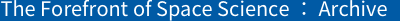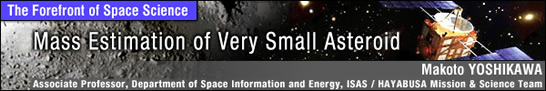| 1 | 2 | 3 |

Estimation of Itokawa’s mass

The mass of an asteroid is important for explorer operation around it. It is also important as a most fundamental value for science. If we can know the mass and volume of asteroids, we can compute their density. In some cases, we can identify substances composing asteroids and their internal structure, etc., from density. The rough shape of Itokawa was known in advance because of the radar observations. Based on observations, its mass was also estimated. For example, Scheeres et al., University of Michigan, reported that Itokawa’s mass is 6.27x1010kg. They calculated this value assuming that the volume of Itokawa is 2.41x107m3 and its density is 2.6g/cm3. Since they used a typical value of asteroids for density, there is no proof that their estimate is correct. Thus, attempts to assess Itokawa’s mass started just after HAYABUSA arrived there.

As stated above, in the initial phase HAYABUSA moved one-dimensionally in an up-down direction to Itokawa. Accordingly, its acceleration can be calculated with a knowledge of high-school physics. Since the acceleration is produced by two forces (solar-radiation pressure and Itokawa’s gravity), they must be separated in order to identify the asteroid’s mass. The separation method is simple. First, we measure acceleration values at different altitudes. Then, assuming that the acceleration generated by solar-radiation pressure is constant, we can calculate acceleration caused by the asteroid’s gravity. In practice, due to other factors such as changes in distance to the Sun and slight attitude changes of the explorer, we need to correct them in the calculation. At this stage we thought that the determination of Itokawa’s mass would be relatively easy. In fact, based on the data acquired for the period from arrival to early October, we were able to calculate Itokawa’s mass to an error margin of 15% for the finally determined value.

Thus, we expected that the accuracy of the estimate of the asteroid’s mass would improve as HAYABUSA gradually neared Itokawa. An unforeseen accident occurred, however. In early October, one of the reaction wheels to control the explorer’s attitude failed. Three reaction wheels were originally onboard HAYABUSA, but one of them malfunctioned before its arrival at Itokawa. Because of this additional failure, we had to use thrusters (chemical engines) to control the explorer’s attitude. The problem is that the thrusters inevitably create acceleration in orbital motion. Thus, it became difficult to estimate Itokawa’s mass based on HAYABUSA’s orbital data.

As a solution, we decided to set certain periods on October 21 and 22 when no attitude control would be performed in order to estimate Itokawa’s mass based on the movement of HAYABUSA during the period. In addition, in November, we conducted several descents to Itokawa including touchdowns. In the second rehearsal descent conducted on November 12, we estimated the asteroid’s mass using the descent orbit. These mass estimates were made without use of range and Doppler data. Instead, we used data of the distance from Itokawa’s surface measured by LIDAR and photographs of the asteroid to determine HAYABUSA’s accurate orbit and simultaneously to calculate Itokawa’s mass. In the second rehearsal descent, in particular, the mass was estimated for the two periods under the initiative of the Kobe University’s LIDAR team. Fig. 4 shows the orbital profiles when the mass was estimated. It shows that HAYABUSA flew close by the asteroid. Itokawa’s distribution of matter (i.e., Itokawa’s shape) was considered in these estimations; it was not treated as a single mass point.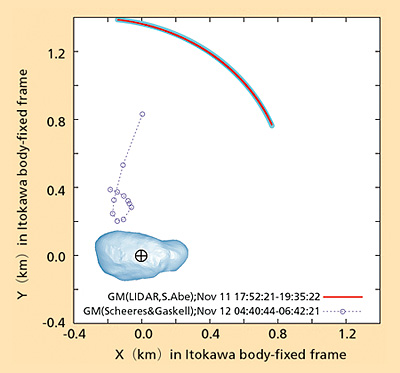Figure 4. Two paths from Nov. 11 to 12 wherein mass estimation was performed during the descent In the Nov. 11 data, the light blue solid line shows measured values while the red line shows the obtained orbit. In the Nov. 12 data, the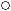marks show measured value while the dotted line shows the obtained orbit. They correspond to C and D in the Table respectively. The figure was produced by Shinsuke Abe (Kobe University) and Daniel Scheeres (University of Michigan). The shape of Itokawa is according to the Aizu shape model, Ver. 5.04.

The mass estimates above are summarized in the Table. These results were obtained by different techniques for different paths by different independent groups. The results coincide within the error range, and Itokawa’s estimated mass is the weighted mean of these values, 3.51x1010kg. From the aspect of science, density is more focused than mass. An accurate estimate of the volume of an object is required to calculate its density. According to the Aizu model for shape, Itokawa’s volume is estimated at 1.84x107m3. Itokawa’s density is accordingly about 1.9g/cm3. This value is considerably smaller than that of other same-type asteroids and, therefore, is important information for further study of Itokawa’s structure or origin.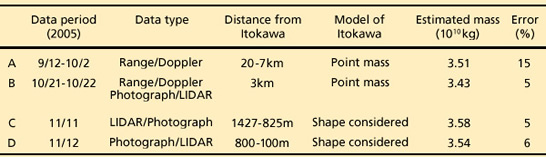Table. Results of Itokawa’s mass estimation

Toward further analysis

The above outlines the initial analysis of Itokawa’s mass. With more precise analysis of HAYABUSA’s orbit in the future, more exact findings on the asteroid’s mass can be revealed. This is our first attempt to estimate the mass of a small asteroid such as Itokawa. Initially, we had some concerns on this challenge that, for example, it might be impossible to identify the mass without the explorer circling around Itokawa or it might be difficult to separate solar-radiation pressure. These were, in fact, not big problems. Instead, we found that the major obstacle for mass estimation was disturbance to the explorer’s orbital motion caused by orbital and attitude control. We will proceed with further data analysis of HAYABUSA. We also expect to have an opportunity to explore other asteroids in the future.

(Makoto YOSHIKAWA)

 | 1 | 2 | 3 |